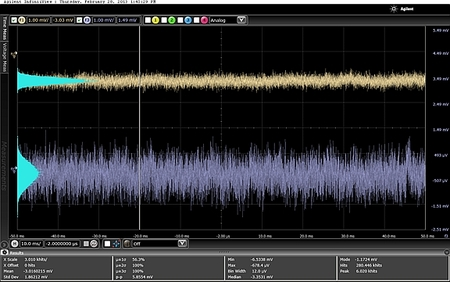I recently heard a claim that at the output of a power converter, digital and analog loop are the same. The claim went like this:

“a power supply using digital power control techniques will appear identical to the end user as a power supply using analog power control techniques.”

This reminds me of the classic interview question about Thevenin and Norton equivalent circuits. It goes like this:

There are two boxes, box A and box B, with the following circuits in them:Box B

How can you determine which is which?

The novice reasons that they both output the same voltage. They both have the same source impedance. When they give up, you tell them that one of them consumes more power and heats up. The hot one contains the Norton circuit.

I have another great interview question:

There are two boxes, box A and box B, with the following circuits in them:Box ABox B

One box has a power converter with a Digital Control Loop, and the other box has a power converter with an Analog Control loop. They both output 1V with identical output impedance at all frequencies, and they have identical transient behavior. How do you determine which box is which?

The answer is quite simple, put a scope on each box and compare the quantization noise.

The Digital Control Loop uses an ADC to capture output voltage and/or current, a Finite Math Compensator, and a Digital PWM. The ADC, Compensator, and PWM all have finite resolution. The Digital Control Loop is therefore a sampled and quantized data system. The Analog Control Loop uses a Ramp and Comparator with Analog Compensator, which is also a sampled data system, but it is not a quantized system. There is only a very low level of noise similar to noise in Op Amps.

The Digital Control Loop will have quantization noise, and the Analog Control Loop will not, therefore we can tell which box is which.

For example, I compared a Digital and Analog Loop using the same PWM clock rate, same output filter design, same compensation, and same FET switches. The load transient of these two loops is identical.

On the scope here is what you see in 100ms of data.The upper trace is the Analog Loop, and the lower trace is the Digital Loop. You can visually see lower frequency affects in the middle of the digital loop waveform and you can also see it riding on the peaks of the switching noise.

A histogram shows the probability distribution of the voltage:The results look similar. However, if you apply a low pass filter to the waveform you can see a big difference:The analog waveform has a much tighter distribution.

Now lets pull the data into a spreadsheet and have a closer look. I have normalized the histogram and plotted the Probability Distribution Function (PDF).The two PDFs are very similar, because the switching noise is similar, due to the using the same switches and output filter. However, a filtered PDF shows a significant difference in the distribution.The Analog Loop produces a much tighter distribution than the Digital Loop, because it lacks quantization noise.

With some spreadsheet work, the unfiltered waveforms have about -46dB of RMS noise. The filtered waveforms have about -60dB and -70dB of RMS noise.

Digital 0.00496 -46.1DB

Analog 0.00450 -46.9DB

Digital 0.00093 -60.1DB

Analog 0.00028 -70.1DB

The distribution of the low frequency content is different. The Analog Loop has 10dB (3X) lower noise spreading in the low frequencies. The 3X narrower PDF is what your eyes see in the scope display in the dark band in the middle of the Analog Loop waveform.

Therefore, like the Thevenin and Norton equivalents, they are not exactly the same. The Norton equivalent produces heat, and the Digital Loop produces a wider distribution of noise.

So next time you throw the Thevenin and Norton equivalent at a smart engineering graduate, if the correct answer rolls smugly off their tongue, consider tossing this question at them and see how they do.